|

# 找准时机把好关键,监理揪出了多少家装“致命伤”!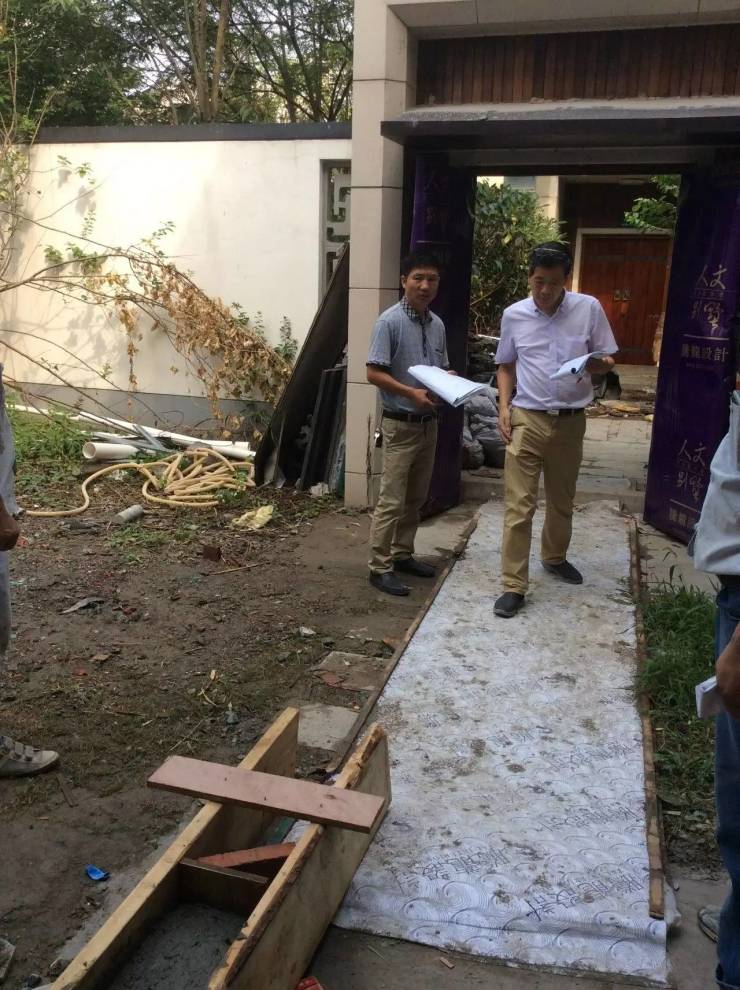▲第1个监理必须到场的节点：修材料进场验收，不管是装修公司提供的材料还是装修业主自主采购的材料进场，各位读者较好都要亲自到工地进行材料验收，主要看材料是不是和合同规定的相同，着重检查材料质量和核对数量。▲第2个监理必须到场的节点：每项工序完成，水电改造结束之后的验收是重中之重，改造的水管要进行打压试验，水压保持1小时不掉压或平均掉压0.05兆帕才合格。电路里的照明电线需要横截面积为2.5平方毫米，空调、电冰箱等家用电器需要使用横截面积为4平方毫米以上的独立回路电线，电路验收时应做通电试验等。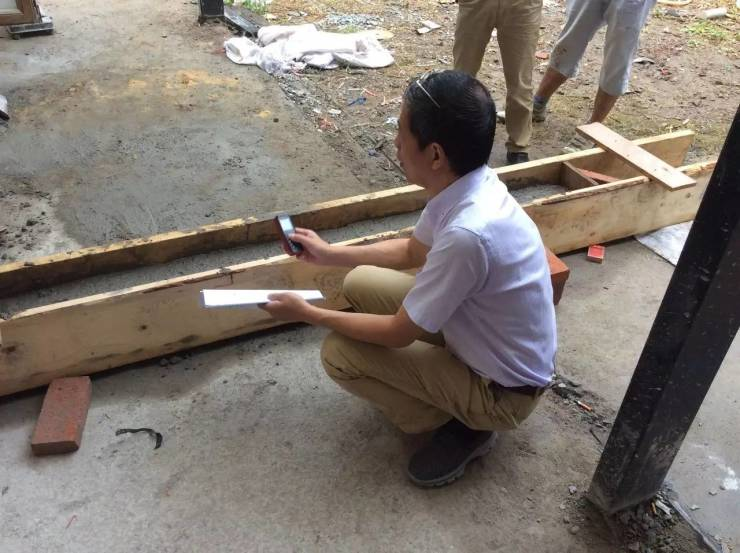▲第3个监理必须到场的节点：墙面检查，这里指的是在刷漆或贴砖之前，主要验看瓦工对墙面有没有处理到位。比如老房的墙面，在刷漆前有没有把基底处理干净；贴砖之前有没有做拉毛处理。这些都对日后墙面的牢固度和瓷砖空鼓率有很大影响。▲第4个监理必须到场的节点：竣工验收，是综合性验收，验收的主要是地砖、墙砖的空鼓率、油漆是否光洁、木作的门开关是否顺畅、有没有缝隙、地板是否有响声等。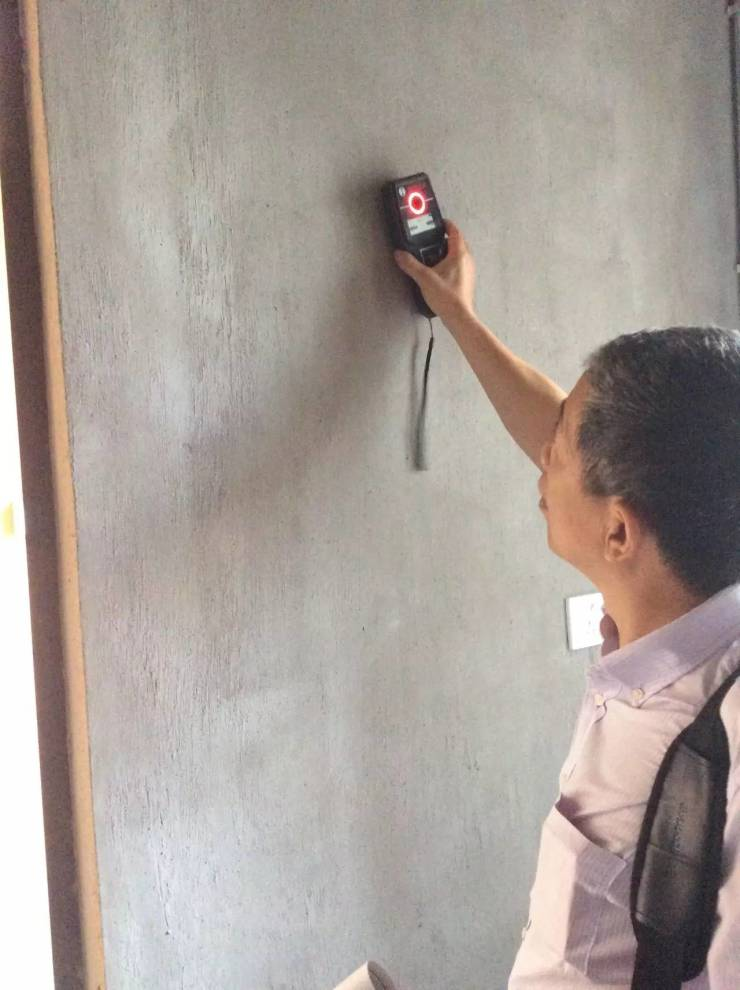▲监理验收墙壁：近年来，墙体质量也成为装修前期的重要验收标准。在新房交付前，下过大雨的第二天前往视察一下。这时候墙壁如果有问题，几乎是无可遁形的。墙壁除了渗水外，此外，也需要验收是否有存在裂纹。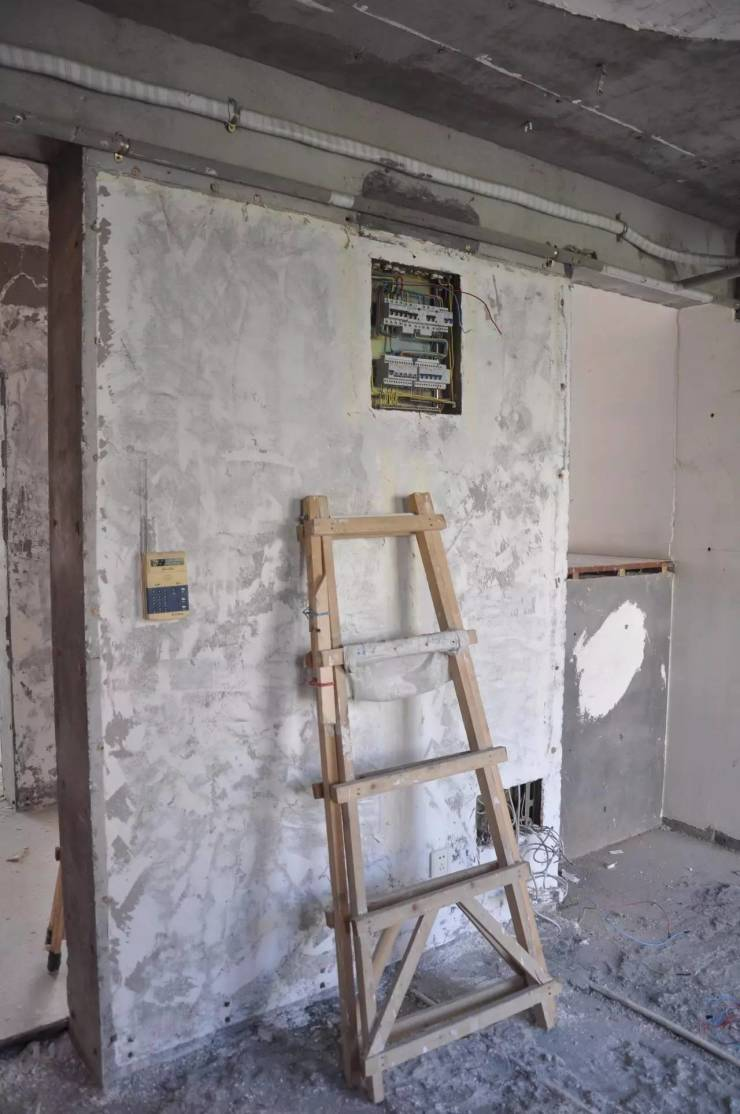▲注意墙顶是否有打眼的地方，有些开发商会告知是预埋件，其实应该是楼上漏水了，为了排出楼板中的水而使用的方式，也就是楼上与楼下没有很好的防水性。用尺子拉下四个边，这样可以知道室内是否是斜的，因为如果是斜房很有可能在装修和摆放家具时有不同的缝隙。▲监理验收水电：验一下房屋的水电是否通了。当然，多数的水管、电线后期都要更换，所以有时候这些内容倒不是关键的，但如果您不打算更换水管、电线的话，那么这些东西就必须认真验收了。▲其次是验收电路，除了看看是否通电外，主要是看电线是否符合国家标准、电线的截面面积是否符合要求。一般来说，家里的电线不应低于2.5mm⊃2;,空调线更应达到4mm⊃2;，否则使用空调时，容易过热变软。▲监理验收防水：这里所说的防水，指的是厨卫的防水。如果在交付时已经做了防水处理，那么就需要对防水质量来验证。如果装修前不试一试，在您装修好后再发现漏水，那么维护工程就大了。不得不拆除已经装修一新的地面来做一层新的防水层。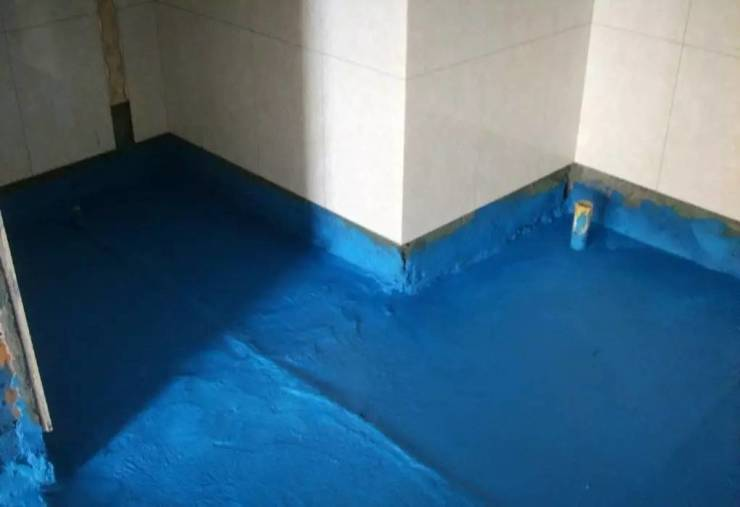▲验收防水的办法是比较常见，用水泥沙浆做一个槛堵着厨房、卫生间的门口，拿一胶袋罩着排污或排水口并捆实，然后在厨房、卫生间地面上放水，浅浅的就行了(水高约50px)。约好楼下的业主，在24小时后查看其家厨房、卫生间的天花是否有渗水。▲监理验收管道：这里所指的管道，是排水或排污管道，尤其是阳台等处的排污口。验收时，拿一个盛水的器具，倒水进排水口，看看水是不是顺利地流走。有些施工人员在清洁时往往会把一些水泥渣倒进排水管，如果这些水泥较黏的话，就会弯头处堵塞，造成排水困难。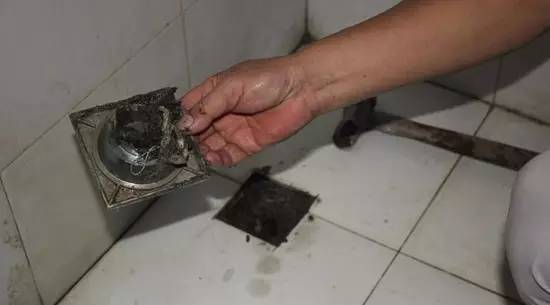▲还有一种情况，就是看看排污管是否有蓄水防臭弯头。按照经验，如果排污管没有蓄水防臭弯头，那么整体房屋质量也就得打折扣了。要知道，防臭地漏远远不能满足实际需要。这种小地方，往往最能体现建筑商的施工质量。▲监理验收地平：验地平就是测量一下离门口最远的室内地面与门口内地面的水平误差。很多时候也可以体现发展商的建筑质量。作为读者，大家根本不可能去验收主体结构，那么就只能从这些细节来看质量了。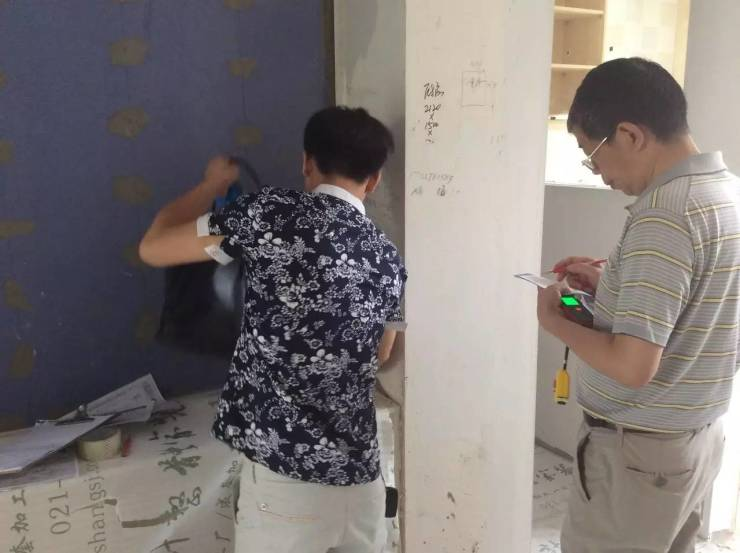▲监理验收层高：如果合同有这—条款。那么您就应该测量一下楼的层高。方法很简单。把尺顺着其中两堵墙的阴角测量。一般来说，层高2.65m左右是可接受的范围；如果低于2.6m，就得考虑了，这种房屋日后不得不生活在一种压抑的环境里。而对于发展商来说，是一种非常有效的节约成本的方法。▲监理验收门窗：这里尤其以验收窗为主。验收的关键是窗和阳台门的密封性。窗的密封性验收最麻烦的一点是，只有在大雨天方能试出好坏，但一般可以通过查看密封胶条是否完整、牢固这一点来证实。▲监理验收其他项目：事实上， 装修施工过程一般要持续两三个月，很多排名前列次装修的读者，怕工地出现猫腻，恨不得天天站在工地监工，非常累！如果你想减轻自己的负担，不妨找一位靠谱的监理来提供全程服务吧！

`声明：本文由入驻焦点开放平台的作者撰写，除焦点官方账号外，观点仅代表作者本人，不代表焦点立场错误信息举报电话： 400-099-0099，邮箱：jubao@vip.sohu.com，或点此进行意见反馈，或点此进行举报投诉。`A B C D E F G H J K L M N P Q R S T W X Y Z
A - B - C - D - E
• A
• 鞍山
• 安庆
• 安阳
• 安顺
• 安康
• 澳门
• B
• 北京
• 保定
• 包头
• 巴彦淖尔
• 本溪
• 蚌埠
• 亳州
• 滨州
• 北海
• 百色
• 巴中
• 毕节
• 保山
• 宝鸡
• 白银
• 巴州
• C
• 承德
• 沧州
• 长治
• 赤峰
• 朝阳
• 长春
• 常州
• 滁州
• 池州
• 长沙
• 常德
• 郴州
• 潮州
• 崇左
• 重庆
• 成都
• 楚雄
• 昌都
• 慈溪
• 常熟
• D
• 大同
• 大连
• 丹东
• 大庆
• 东营
• 德州
• 东莞
• 德阳
• 达州
• 大理
• 德宏
• 定西
• 儋州
• 东平
• E
• 鄂尔多斯
• 鄂州
• 恩施
F - G - H - I - J
• F
• 抚顺
• 阜新
• 阜阳
• 福州
• 抚州
• 佛山
• 防城港
• G
• 赣州
• 广州
• 桂林
• 贵港
• 广元
• 广安
• 贵阳
• 固原
• H
• 邯郸
• 衡水
• 呼和浩特
• 呼伦贝尔
• 葫芦岛
• 哈尔滨
• 黑河
• 淮安
• 杭州
• 湖州
• 合肥
• 淮南
• 淮北
• 黄山
• 菏泽
• 鹤壁
• 黄石
• 黄冈
• 衡阳
• 怀化
• 惠州
• 河源
• 贺州
• 河池
• 海口
• 红河
• 汉中
• 海东
• 怀来
• I
• J
• 晋中
• 锦州
• 吉林
• 鸡西
• 佳木斯
• 嘉兴
• 金华
• 景德镇
• 九江
• 吉安
• 济南
• 济宁
• 焦作
• 荆门
• 荆州
• 江门
• 揭阳
• 金昌
• 酒泉
• 嘉峪关
K - L - M - N - P
• K
• 开封
• 昆明
• 昆山
• L
• 廊坊
• 临汾
• 辽阳
• 连云港
• 丽水
• 六安
• 龙岩
• 莱芜
• 临沂
• 聊城
• 洛阳
• 漯河
• 娄底
• 柳州
• 来宾
• 泸州
• 乐山
• 六盘水
• 丽江
• 临沧
• 拉萨
• 林芝
• 兰州
• 陇南
• M
• 牡丹江
• 马鞍山
• 茂名
• 梅州
• 绵阳
• 眉山
• N
• 南京
• 南通
• 宁波
• 南平
• 宁德
• 南昌
• 南阳
• 南宁
• 内江
• 南充
• P
• 盘锦
• 莆田
• 平顶山
• 濮阳
• 攀枝花
• 普洱
• 平凉
Q - R - S - T - W
• Q
• 秦皇岛
• 齐齐哈尔
• 衢州
• 泉州
• 青岛
• 清远
• 钦州
• 黔南
• 曲靖
• 庆阳
• R
• 日照
• 日喀则
• S
• 石家庄
• 沈阳
• 双鸭山
• 绥化
• 上海
• 苏州
• 宿迁
• 绍兴
• 宿州
• 三明
• 上饶
• 三门峡
• 商丘
• 十堰
• 随州
• 邵阳
• 韶关
• 深圳
• 汕头
• 汕尾
• 三亚
• 三沙
• 遂宁
• 山南
• 商洛
• 石嘴山
• T
• 天津
• 唐山
• 太原
• 通辽
• 铁岭
• 泰州
• 台州
• 铜陵
• 泰安
• 铜仁
• 铜川
• 天水
• 天门
• W
• 乌海
• 乌兰察布
• 无锡
• 温州
• 芜湖
• 潍坊
• 威海
• 武汉
• 梧州
• 渭南
• 武威
• 吴忠
• 乌鲁木齐
X - Y - Z
• X
• 邢台
• 徐州
• 宣城
• 厦门
• 新乡
• 许昌
• 信阳
• 襄阳
• 孝感
• 咸宁
• 湘潭
• 湘西
• 西双版纳
• 西安
• 咸阳
• 西宁
• 仙桃
• 西昌
• Y
• 阳泉
• 运城
• 营口
• 盐城
• 扬州
• 鹰潭
• 宜春
• 烟台
• 宜昌
• 岳阳
• 益阳
• 永州
• 阳江
• 云浮
• 玉林
• 宜宾
• 雅安
• 玉溪
• 延安
• 榆林
• 银川
• Z
• 张家口
• 镇江
• 舟山
• 漳州
• 淄博
• 枣庄
• 郑州
• 周口
• 驻马店
• 株洲
• 张家界
• 珠海
• 湛江
• 肇庆
• 中山
• 自贡
• 资阳
• 遵义
• 昭通
• 张掖
• 中卫

1室1厅1厨1卫1阳台

1
2
3
4
5

0
1
2

1

1

0
1
2
3报名成功，资料已提交审核A B C D E F G H J K L M N P Q R S T W X Y Z
A - B - C - D - E
• A
• 鞍山
• 安庆
• 安阳
• 安顺
• 安康
• 澳门
• B
• 北京
• 保定
• 包头
• 巴彦淖尔
• 本溪
• 蚌埠
• 亳州
• 滨州
• 北海
• 百色
• 巴中
• 毕节
• 保山
• 宝鸡
• 白银
• 巴州
• C
• 承德
• 沧州
• 长治
• 赤峰
• 朝阳
• 长春
• 常州
• 滁州
• 池州
• 长沙
• 常德
• 郴州
• 潮州
• 崇左
• 重庆
• 成都
• 楚雄
• 昌都
• 慈溪
• 常熟
• D
• 大同
• 大连
• 丹东
• 大庆
• 东营
• 德州
• 东莞
• 德阳
• 达州
• 大理
• 德宏
• 定西
• 儋州
• 东平
• E
• 鄂尔多斯
• 鄂州
• 恩施
F - G - H - I - J
• F
• 抚顺
• 阜新
• 阜阳
• 福州
• 抚州
• 佛山
• 防城港
• G
• 赣州
• 广州
• 桂林
• 贵港
• 广元
• 广安
• 贵阳
• 固原
• H
• 邯郸
• 衡水
• 呼和浩特
• 呼伦贝尔
• 葫芦岛
• 哈尔滨
• 黑河
• 淮安
• 杭州
• 湖州
• 合肥
• 淮南
• 淮北
• 黄山
• 菏泽
• 鹤壁
• 黄石
• 黄冈
• 衡阳
• 怀化
• 惠州
• 河源
• 贺州
• 河池
• 海口
• 红河
• 汉中
• 海东
• 怀来
• I
• J
• 晋中
• 锦州
• 吉林
• 鸡西
• 佳木斯
• 嘉兴
• 金华
• 景德镇
• 九江
• 吉安
• 济南
• 济宁
• 焦作
• 荆门
• 荆州
• 江门
• 揭阳
• 金昌
• 酒泉
• 嘉峪关
K - L - M - N - P
• K
• 开封
• 昆明
• 昆山
• L
• 廊坊
• 临汾
• 辽阳
• 连云港
• 丽水
• 六安
• 龙岩
• 莱芜
• 临沂
• 聊城
• 洛阳
• 漯河
• 娄底
• 柳州
• 来宾
• 泸州
• 乐山
• 六盘水
• 丽江
• 临沧
• 拉萨
• 林芝
• 兰州
• 陇南
• M
• 牡丹江
• 马鞍山
• 茂名
• 梅州
• 绵阳
• 眉山
• N
• 南京
• 南通
• 宁波
• 南平
• 宁德
• 南昌
• 南阳
• 南宁
• 内江
• 南充
• P
• 盘锦
• 莆田
• 平顶山
• 濮阳
• 攀枝花
• 普洱
• 平凉
Q - R - S - T - W
• Q
• 秦皇岛
• 齐齐哈尔
• 衢州
• 泉州
• 青岛
• 清远
• 钦州
• 黔南
• 曲靖
• 庆阳
• R
• 日照
• 日喀则
• S
• 石家庄
• 沈阳
• 双鸭山
• 绥化
• 上海
• 苏州
• 宿迁
• 绍兴
• 宿州
• 三明
• 上饶
• 三门峡
• 商丘
• 十堰
• 随州
• 邵阳
• 韶关
• 深圳
• 汕头
• 汕尾
• 三亚
• 三沙
• 遂宁
• 山南
• 商洛
• 石嘴山
• T
• 天津
• 唐山
• 太原
• 通辽
• 铁岭
• 泰州
• 台州
• 铜陵
• 泰安
• 铜仁
• 铜川
• 天水
• 天门
• W
• 乌海
• 乌兰察布
• 无锡
• 温州
• 芜湖
• 潍坊
• 威海
• 武汉
• 梧州
• 渭南
• 武威
• 吴忠
• 乌鲁木齐
X - Y - Z
• X
• 邢台
• 徐州
• 宣城
• 厦门
• 新乡
• 许昌
• 信阳
• 襄阳
• 孝感
• 咸宁
• 湘潭
• 湘西
• 西双版纳
• 西安
• 咸阳
• 西宁
• 仙桃
• 西昌
• Y
• 阳泉
• 运城
• 营口
• 盐城
• 扬州
• 鹰潭
• 宜春
• 烟台
• 宜昌
• 岳阳
• 益阳
• 永州
• 阳江
• 云浮
• 玉林
• 宜宾
• 雅安
• 玉溪
• 延安
• 榆林
• 银川
• Z
• 张家口
• 镇江
• 舟山
• 漳州
• 淄博
• 枣庄
• 郑州
• 周口
• 驻马店
• 株洲
• 张家界
• 珠海
• 湛江
• 肇庆
• 中山
• 自贡
• 资阳
• 遵义
• 昭通
• 张掖
• 中卫• 手机• 分享
• 设计
免费设计
• 计算器
装修计算器
• 联系
• 置顶
返回顶部